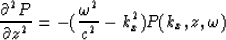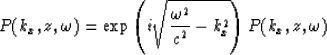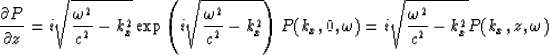Next: ELIMINATION OF EVANESCENT ENERGY Up: Ji: generalized phase-shift method Previous: Tal-Ezer Method

# GENERATION OF SURFACE VALUES OF P ANDThe initiation of migration based on equation (4) requires the specification of both P andon the surface. Since only one of these fields is recorded in practice, the remaining field must be generated from mathematical assumptions. In a region in which the acoustic velocity c is constant, the acoustic wave equation can be doubly transformed in x and t to give(20)
where P is the two dimensionally transformed pressure and kx is the horizontal wavenumber. The solution to equation (20) are given by(21)
the solution (21) includes only upgoing waves under the convention that z increases with depth. The doubly transformed pressure gradients can be obtained from equation (21) by differentiation(22)
The generated vertical pressure gradient on the surface are obtained from equation (22) by setting z=0 and by an inverse Fourier transformation with respect to x.Next: ELIMINATION OF EVANESCENT ENERGY Up: Ji: generalized phase-shift method Previous: Tal-Ezer Method
Stanford Exploration Project
1/13/1998